Courses
Courses for Kids
Free study material
Free LIVE classes
More

# Gravitation Potential Energy for JEELIVE
Join Vedantu’s FREE Mastercalss

## What is Gravity?

There are many discoveries to Newton's gravitational theories. So now we calculate g, the gravitational force with the distance between the mass, m, and the Earth, which is the Earth's radius. To say it in a straightforward manner, we consider that the centre of the Earth contains all of its mass. This might seem more reasonable when the object is at a far distance from Earth, where the radius of the Earth is negligible, but not when on the Earth's surface. However, this assumption is accurate for any object that isn't in a gravitational sphere. As it's known that all conservative forces acquire potential energy related to them, and it is no exception for a force of gravity.

## Understanding Gravitational Potential

Before diving into what is the equation for gravitational potential due to a spherical shell, it's essential to understand gravitational potential. The effort made against gravity to bring a mass from infinity to a point is known as gravitational potential. Then to define what is gravitational potential energy, it can be said that the amount of work required to displace a body of mass (m) from infinity to a position inside the gravitational field of a source mass (M) without any acceleration, the work is stored as potential energy. This has the symbol Ug

The change in potential energy is equivalent to the amount of work performed on the body by the forces if the position of the body changes as a result of the application of external forces. The work performed under the influence of gravitational force is independent of the approach used for a change in position, making it a conservative force. Additionally, all of these forces have some potential. So what is a reference point? It is defined as the point where potential energy is zero because the gravitational force on a body at infinity is zero.

## Derivation of the Formula of Gravitational Potential Energy

The standard equation for gravitational potential energy is denoted by,

$V=mgh$

where the m is mass,

g is the acceleration due to gravity, and

h refers to the height above the ground.

Let's derive the gravitational potential formula.

Consider the mass of the Earth is "M." Take a point "P" where the gravitational potential energy is required to be calculated at a distance of 'r,' which is greater than 'R,' the radius of Earth. Again, take another point, "A," at a distance 'x' from the centre of the Earth, and let x be greater than r. Consider the unit mass to be placed at point "A," so the acting force would be,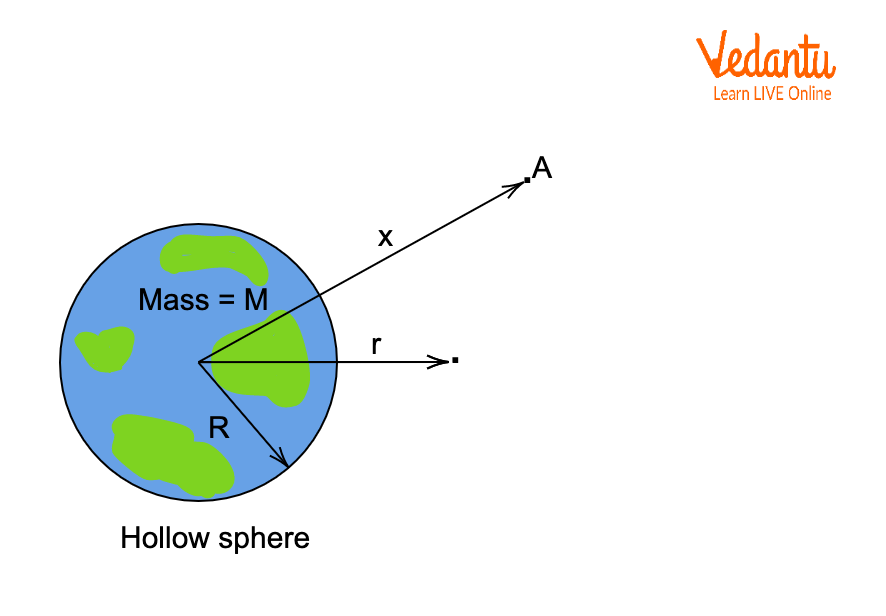Gravitational Potential Energy

$\text{F}=\frac{GMm}{{{x}^{2}}}$

$=\frac{GM(1)}{{{x}^{2}}}$

$=\frac{GM}{{{x}^{2}}}$

When the mass m is moved from A to B without any acceleration via a small distance, 'dx,' the work expression would be

$\text{dW}=\text{F}\cdot \text{dx}$

$=\frac{GM}{{{x}^{2}}}\cdot dx$

Doing the integration, the work equation would be,

$W=-\frac{GM}{r}$

The work is the expression for gravitational potential energy at that point,

$V=-\frac{GM}{r}$

Here, G is the universal gravitational constant

M represents the mass of Earth.

Note that the gravitational potential is negative all the time at a point and reaches its highest value at infinity. The unit of gravitational potential energy is J/Kg in the SI system. The dimension of the equation of gravitational potential is [M0L2T-2].

Now let's derive the relation between gravitational field intensity and gravitational potential. Expressing the equation in integral form would be,

$V=-\int \vec{E} \cdot \overrightarrow{d r}$

In case the value of E is given in the context of finding V, this gravitational potential energy equation can be applied. But in case V is given to find the E value, the differential form of the equation is applied.

$\vec{E}=\frac{\partial V}{\partial x} \hat{i}+\frac{\partial V}{\partial y} \hat{j}+\frac{\partial V}{\partial z} \hat{k}$

## Calculating the Gravitational Potential due to a Spherical Shell

In order to obtain the formula for gravitational potential due to a spherical shell, there can be two cases considered, as the spherical shell can be solid or hollow.

• For the solid sphere, consider the point is present outside of the sphere when r > R. Then the expression would be $V=-\frac{GM}{r}$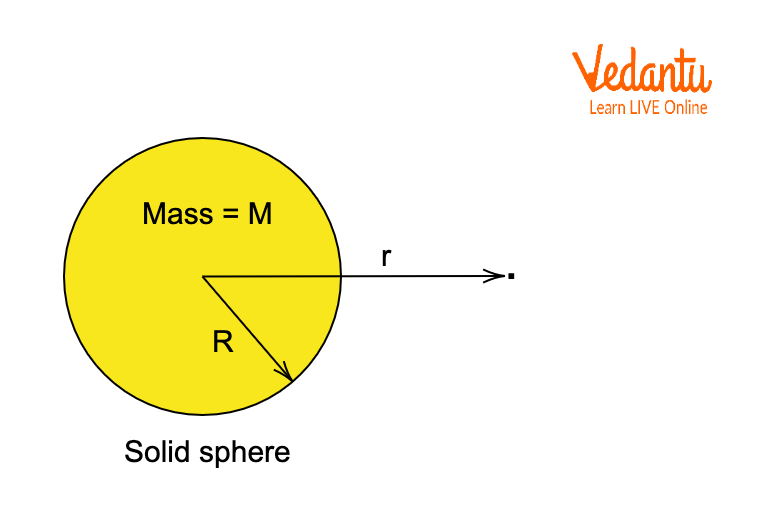Solid Sphere with r > R

• When the point is on the sphere, with r = R, $V=-\frac{GM}{R}$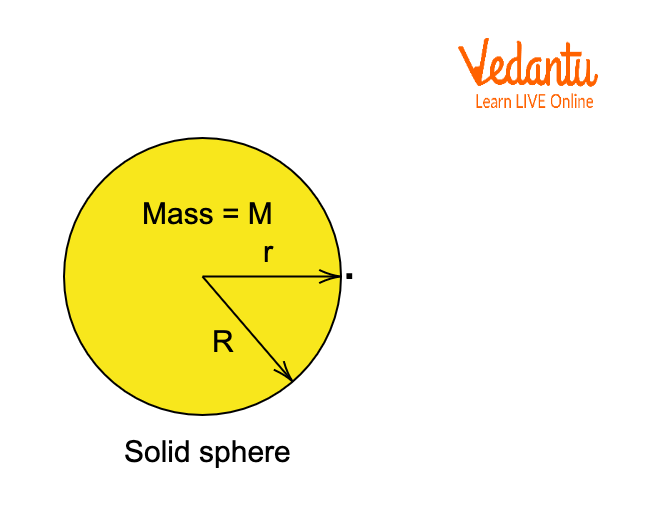Solid Sphere with r = R

• When the point is inside the sphere, with r < R, $V=-G M\left[\frac{3 R^{2}-r^{2}}{2 R^{3}}\right]$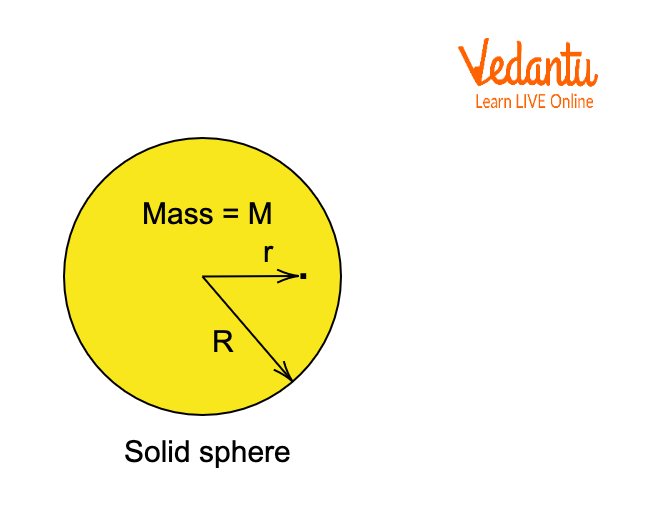Solid Sphere with r < R

• When the point is at the centre of the sphere, r = 0, $V=-\frac{3}{2}\left(\frac{G, M}{R}\right).$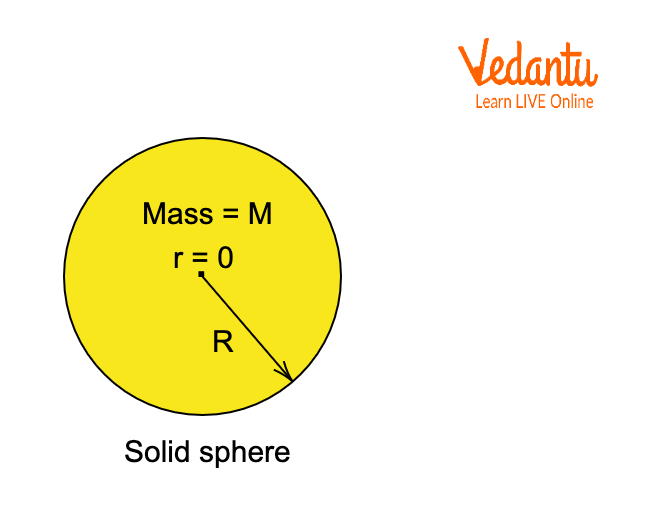Solid Sphere with r = 0

• In the case of a hollow sphere, if the point is outside of the shell, r > R, then $V=-\frac{GM}{r}$Hollow Sphere with r > R

• When the point lies on the surface, r= R, then $V=-\frac{GM}{R}$Hollow Sphere with r = R

• When the point is inside the shell, r < R,  then $V=-\frac{GM}{R}$.Hollow Sphere with r < R

## Conclusion

The above study explains many cases of gravitational potential energy derivation. Additionally, the article also throws light on how the expression changes applied to many cases with a spherical shell. Students who are appearing on JEE Main can refer to these explanations to understand gravitational potential energy.

Last updated date: 23rd Sep 2023
Total views: 124.2k
Views today: 2.24k

## FAQs on Gravitation Potential Energy for JEE

1. What is the gravitational potential?

The quantity of work done by displacing an object with unit mass from infinity to the point in the gravitational field is known as the gravitational potential energy. The gravitational potential energy is represented by V. The expression of gravitational potential energy is

$V=\frac{-GMm}{r}$

where M is the mass placed in the direction of the x-axis, m is the test mass at infinity, and r is the distance from the source mass where the gravitational potential energy is calculated. J/kg is the unit of gravitational energy.

2. What is gravitational Self-Energy?

In order to define the gravitational self-energy of a system of particles or a body, the work is considered, which is done by an external agent for assembling the body or the system of particles from the infinitesimal elements which were initially separated by the infinite distance apart. The expression is

$U_s=-\frac{1}{2} G n(n-1) \frac{m^{2}}{r}$

Here,

• Us is gravitational self-energy,

• G is a constant or universal gravitational constant,

• n is the number of particles or bodies,

• m is the mass of each body or particle, and

• r is the average distance between two particles.